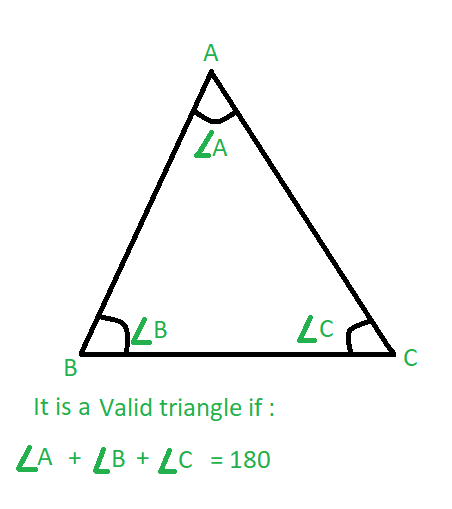# Check whether the triangle is valid or not if angles are given

Given three integers A, B and C which are the three angles of a possible triangle in degrees, the task is to check whether the triangle is valid or not.

Examples:

Input: A = 60, B = 40, C = 80
Output: Valid

Input: A = 55, B = 45, C = 60
Output: Invalid

## Recommended: Please try your approach on {IDE} first, before moving on to the solution.

Approach: A triangle is valid if the sum of the three angles is equal to 180 degrees.Below is the implementation of the above approach:

## C++

 `// C++ implementation of the approach ` `#include ` `using` `namespace` `std; ` ` `  `// Function to check if sum of the ` `// three angles is 180 or not ` `bool` `Valid(``int` `a, ``int` `b, ``int` `c) ` `{ ` `    ``// Check condition ` `    ``if` `(a + b + c == 180 && a != 0 && b != 0 && c != 0) ` `        ``return` `true``; ` `    ``else` `        ``return` `false``; ` `} ` ` `  `// Driver code ` `int` `main() ` `{ ` `    ``int` `a = 60, b = 40, c = 80; ` ` `  `    ``if` `(Valid(a, b, c)) ` `        ``cout << ``"Valid"``; ` `    ``else` `        ``cout << ``"Invalid"``; ` `} `

## Java

 `// Java program to check ` `// validity of any triangle ` `class` `GFG { ` ` `  `    ``// Function to check if sum of the ` `    ``// three angles is 180 or not ` `    ``public` `static` `int` `Valid(``int` `a, ``int` `b, ``int` `c) ` `    ``{ ` `        ``// check condition ` `        ``if` `(a + b + c == ``180` `&& a != ``0` `&& b != ``0` `&& c != ``0``) ` `            ``return` `1``; ` `        ``else` `            ``return` `0``; ` `    ``} ` ` `  `    ``// Driver Code ` `    ``public` `static` `void` `main(String args[]) ` `    ``{ ` ` `  `        ``int` `a = ``60``, b = ``40``, c = ``80``; ` ` `  `        ``// function calling and print output ` `        ``if` `((Valid(a, b, c)) == ``1``) ` `            ``System.out.print(``"Valid"``); ` `        ``else` `            ``System.out.print(``"Invalid"``); ` `    ``} ` `} ` ` `  `// This code is contributed ` `// by Apurva Sharma `

## Python3

 `# Python3 implementation of the approach ` ` `  `# Function to check if sum of the ` `# three angles is 180 or not ` `def` `Valid(a, b, c): ` ` `  `    ``# Check condition ` `    ``if` `((a ``+` `b ``+` `c ``=``=` `180``) ``and` `a !``=` `0` `and` `b !``=` `0` `and` `c !``=` `0``): ` `        ``return` `True` `    ``else``: ` `        ``return` `False` ` `  `# Driver code ` `if` `__name__ ``=``=` `"__main__"``: ` `    ``a ``=` `60` `    ``b ``=` `40` `    ``c ``=` `80` `    ``if` `(Valid(a, b, c)): ` `        ``print``(``"Valid"``) ` `    ``else``: ` `        ``print``(``"Invalid"``) ` ` `  `# This code is contributed by ` `# sanjeev2552 `

## C#

 `// C# program to check ` `// validity of any triangle ` `using` `System; ` ` `  `class` `GFG { ` ` `  `    ``// Function to check if sum of the ` `    ``// three angles is 180 or not ` `    ``public` `static` `int` `Valid(``int` `a, ``int` `b, ``int` `c) ` `    ``{ ` `        ``// check condition ` `        ``if` `(a + b + c == 180 && a != 0 && b != 0 && c != 0) ` `            ``return` `1; ` `        ``else` `            ``return` `0; ` `    ``} ` ` `  `    ``// Driver Code ` `    ``public` `static` `void` `Main() ` `    ``{ ` `        ``int` `a = 60, b = 40, c = 80; ` ` `  `        ``// function calling and print output ` `        ``if` `((Valid(a, b, c)) == 1) ` `            ``Console.WriteLine(``"Valid"``); ` `        ``else` `            ``Console.WriteLine(``"Invalid"``); ` `    ``} ` `} ` ` `  `// This code is contributed ` `// by anuj_6 `

Output:

```Invalid
```

Attention reader! Don’t stop learning now. Get hold of all the important DSA concepts with the DSA Self Paced Course at a student-friendly price and become industry ready.

My Personal Notes arrow_drop_upCheck out this Author's contributed articles.

If you like GeeksforGeeks and would like to contribute, you can also write an article using contribute.geeksforgeeks.org or mail your article to contribute@geeksforgeeks.org. See your article appearing on the GeeksforGeeks main page and help other Geeks.

Please Improve this article if you find anything incorrect by clicking on the "Improve Article" button below.

Article Tags :
Practice Tags :

2

Please write to us at contribute@geeksforgeeks.org to report any issue with the above content.